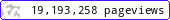•••••MadAsMaths.com :: Maths Booklets :: Basic Topics :: Calculus

BASIC TOPICS - CALCULUS

These booklets are suitable for

• students covering the first year (AS) Calculus material, of a two year course in A Level mathematics.

• revising/introducing Calculus to undergraduate students in degrees not requiring A Level mathematics.

 calculus_introduction_exam_questions.pdfdifferentiation_optimization_problems.pdfdifferentiation_practice_i.pdfintegration_areas_intro.pdfintegration_intro.pdf